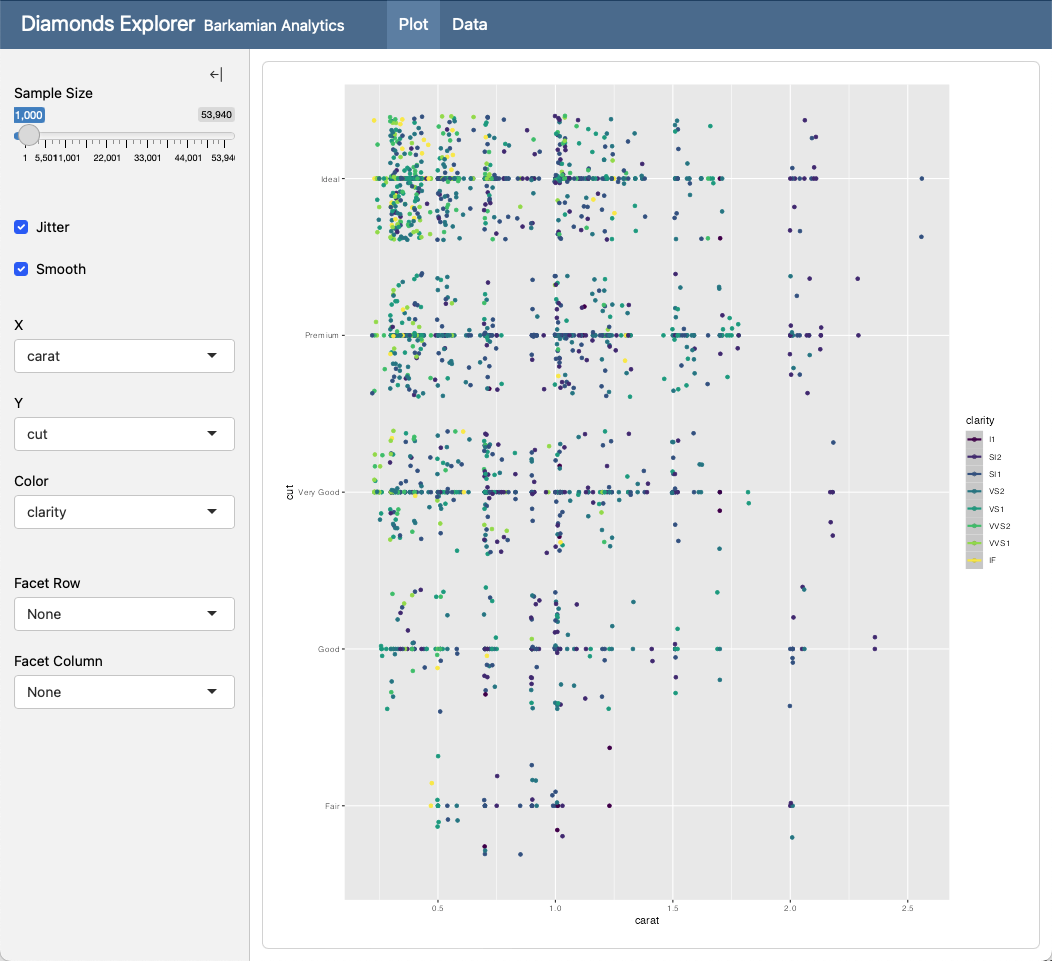# Dashboards with Shiny for R

Pre-release Feature

This feature is new in the upcoming Quarto 1.4 release. To use the feature now, you’ll need to download and install the Quarto pre-release.

## Introduction

The Shiny package provides an easy way to build web applications with R. Quarto dashboards can include embedded Shiny components (e.g. a plot with sliders that control its inputs).

This section covers integrating Shiny with Quarto and assumes that you already have basic familiarity with Shiny. To learn more about Shiny please visit https://shiny.posit.co/r/getstarted/shiny-basics/lesson1/index.html. If you are using Python rather than R, see the documentation on using Shiny for Python.

## Walkthrough

Here we’ll explore an in-depth example that covers many of the techniques you’ll use when creating dashboards with Shiny, including factoring out setup code, reactive calculations, and more advanced layout constructs like sidebars and tabsets. Here is the interactive document we’ll be building:Here is the source code for this dashboard (click on the numbers on the far right for additional explanation of syntax and mechanics):

---
title: "Diamonds Explorer"
author: "Barkamian Analytics"
format: dashboard
server: shiny
---

{r}
#| context: setup
library(ggplot2)
dataset <- diamonds


# {.sidebar}

{r}
sliderInput('sampleSize', 'Sample Size',
min=1, max=nrow(dataset),
value=min(1000, nrow(dataset)),
step=500, round=0)
br()
checkboxInput('jitter', 'Jitter')
checkboxInput('smooth', 'Smooth')


{r}
selectInput('x', 'X', names(dataset))
selectInput('y', 'Y', names(dataset), names(dataset)[])
selectInput('color', 'Color', c('None', names(dataset)))


{r}
selectInput('facet_row', 'Facet Row',
c(None='.', names(diamonds[sapply(diamonds, is.factor)])))
selectInput('facet_col', 'Facet Column',
c(None='.', names(diamonds[sapply(diamonds, is.factor)])))


# Plot

{r}
plotOutput('plot')


# Data

{r}
tableOutput('data')


{r}
#| context: server

dataset <- reactive({
diamonds[sample(nrow(diamonds), input$sampleSize),] }) output$plot <- renderPlot({

p <- ggplot(
dataset(),
aes_string(x=input$x, y=input$y)) + geom_point()

if (input$color != 'None') p <- p + aes_string(color=input$color)

facets <- paste(input$facet_row, '~', input$facet_col)
if (facets != '. ~ .')
p <- p + facet_grid(facets)

if (input$jitter) p <- p + geom_jitter() if (input$smooth)
p <- p + geom_smooth()

p

})

output\$data <- renderTable({
dataset()
})

1
The server: shiny option instructs Quarto to run a Shiny Server behind the document.
2
The context: setup cell option indicates that this code cell should run when the application starts (as opposed to when each new client session starts). Expensive initialization code (e.g. loading data) should be placed in context: setup.
3
Create global sidebars by adding the .sidebar class to level 1 headers. Sidebars can include code cells as well as images, narrative, and links.
4
These select inputs have their contents dynamically driven from the available columns in the dataset.
5
Level 1 headings (here # Plots and # Data) create pages within the dashboard.
6
Include server code (reactives that compute values or render output) in a cell with context: server.
7
The dataset() reactive will be called to re-sample the dataset every time the sample size changes.
8
The renderPlot() function regenerates the plot whenever the dataset() reactive or another input option changes.
9
The renderTable() function regenerates the table whenever the dataset() reactive changes.